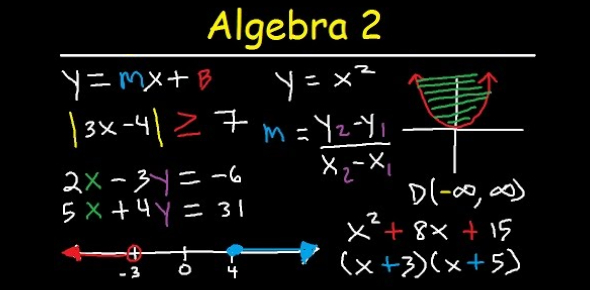# Algebra 2 Semester Exam Practice

36 Questions | Total Attempts: 754SettingsNote: ^ is used to note exponents ie. X^2 reads x to the second power. It is importantthat when entering answers you use spaces between operation symbols. Ex. 2x + 3y instead of 2x+3y And no spaces before and after parenthesis or exponents Ex. 2(x^-2 + 3)

• 1.
Evaluate the given expression if x = 4, y = 8, w = -2 and z = 3.       (x-y)² + 5wz
• A.

-14

• B.

14

• C.

44

• D.

34

• 2.
Simplify  5x + 2y – 7x + y
• 3.
Simplify 6(4x + 8y) + 2(3x – 6y)
• 4.
Simplify (4x² + 3x + 3) + (5x² – 13x – 8).  Use ^ for powers ie. x^2 is x to the second power.
• 5.
Simplify (-10x² – 4x + 8)- (6x² + 10x -3)
• 6.
Simplify  -3xy(6xy³- 4xy + 7y²)
• A.

-18x^2y^4 + 12x^2y^2 - 21xy^3

• B.

-18x^2y^4 - 12x^2y^2 - 21xy^3

• C.

3x^2y^4 - 7x^2y^2 + 4xy^3

• D.

3x^2y^4 + 7x^2y^2 - 4xy^3

• 7.
Simplify 10squrt(162) - squrt(405) - squrt(200)
• 8.
• A.

A

• B.

B

• C.

C

• D.

D

• 9.
• A.

A

• B.

B

• C.

C

• D.

D

• 10.
Solve 24p - 12 = 50; p = _____, round to the nearest hundredth.
• 11.
Solve 5x - 23 = 56; x = ____, round to the nearest tenth.
• 12.
Solve- abs(x - 2) = 27, X = ____
• A.

29

• B.

25

• C.

-25

• D.

29, -25

• 13.
Find the value of f(6) if f(x) = -4x - 2.
• A.

26

• B.

-26

• C.

24

• D.

-24

• 14.
Find the slope of the line that passes through the pair of points (-3,-5) and (7,5).In your answer type the number only.
• 15.
Write an equation in slope-intercept form for the line that satisfies the following condition.  Slope 3, and passes through (5,20).Use spaces between operation symbols when typing your answer.  Ex. y = 3x - 2
• 16.
Solve the system of equations.     x – y = 4 and y = 2x – 6
• A.

X = 2 y = 2

• B.

X = -2 y = 2

• C.

X = -2 y = -2

• D.

X = 2 y = -2

• 17.
Solve the system of equations.    2x + 5y = 12 and x – 3y = -2
• A.

X = 4.8 y = 3.0

• B.

X = 2.4 y = 1.5

• C.

X = 1.2 y = 0.75

• D.

X = 7.2 y = 4.5

• 18.
Describe the system of equation as consistent and independent, consistent and dependent, or none of these.   3x – 2y = 1 and 6x – 9y = 63
• A.

Consistent and independent

• B.

Consistent and dependent

• C.

None of these

• 19.
Express the given number or expression in scientific notation.  Round to the nearest hundredth.  0.0000269074
• 20.
Factor  4xy – 3y – 40x + 30
• A.

(4x - 3)(2y - 20)

• B.

(12x - 9)(2y - 20)

• C.

(y - 10)(4x - 3)

• D.

Y(4x - 3) - 10(4x - 3)

• 21.
Factor 2x² + 3x – 9
• 22.
(5 + ί) + (6 - 12ί)
• A.

11 + 11i

• B.

11 - 11i

• C.

30 - 72i

• D.

11 + 13i

• 23.
(4 - 10ί) + (15 - 16ί)
• 24.
Solve: squrt(2x - 2) + 12 = 14x = ____  Type the number only for your answer.
• 25.
Solve:  squrt(x - 3) + 6 = 15
• A.

64

• B.

84

• C.

74

• D.

34

Related TopicsBack to top